## Deltatron Circuit or Cascaded Modular Voltage Multipliers:

A combination of Cockcroft-Walton type voltage multiplier with cascaded transformer d.c. rectifier is developed recently for very high voltages but limited output currents having higltstability, small ripple factor and fast regulation. One such unit is recently patented by “ENGE” in U.S.A., called “ENGETRON” or `DELTATRON”. The schematic diagram of a typical Deltatron Circuit unit is shown in Fig. 6.5.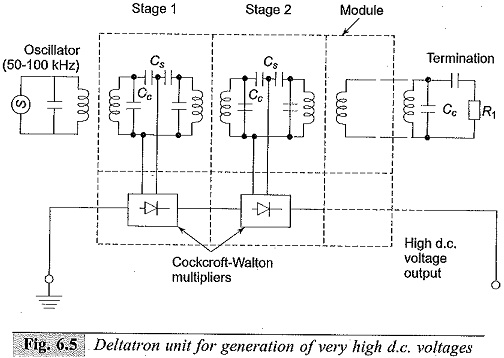Basically this Deltatron Circuit consists of Cockcroft-Walton multiplier units fed from a supply transformer unit (stage 1). These supply transformers are air-cored to have low inductance and are connected in series through capacitors Cs. In addition, the windings of the transformers are shunted by a capacitor Cc to compensate for the magnetizing current. The entire unit is terminated by a load resistor R1. All the Cockcroft-Walton multipliers are connected in series and the entire unit is enclosed in a cylindrical vessel insulated by SF6 gas. Each stage of the unit is typically rated for 10 to 50 kV, and about 20 to 25 stages are used in a unit. The whole assembly is usually of smaller size and weight than a cascaded rectifier unit. The supply frequency to the transformers is from a high frequency oscillator (50 to 100 kHz) and as such the capacitors used are of smaller value. The voltage regulation system is controlled by a parallel R-C divider which in turn controls the supply oscillator. Regulation due to load variations or power source voltage variations is very fast (response time < 1 ms). The disadvantage of this Deltatron Circuit is that the polarity of the unit cannot be reversed easily. Typical units of this type may have a rating of 1 MV, 2 mA with each module or stage rated for 50 kV with ripple content less than 1%.

### Electrostatic Machines:

#### Basic Principle

In electromagnetic machines, current carrying conductors are moved in a magnetic field, so that the mechanical energy is converted into electrical energy. In electrostatic machines charged bodies are moved in an electric field against an electrostatic field in order that mechanical energy is converted into electrical energy. Thus, if an insulated belt with a charge density δ moves in an electric field “E(x)” between two electrodes with separation ‘s’ then

• the charge on the strip of belt at a distance dx is dq = δb.dx where b is the width of the belt, and
• the force on the belt, F is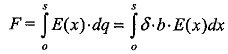If the belt moves with a velocity, ν, then the mechanical power P, required to move the belt is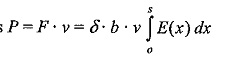The current, I, in the system is given as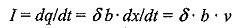and the potential difference, V, between the electrodes is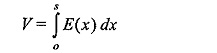Thus, in an electrostatic machine, the mechanical power required to move the belt at a velocity ν, i.e. P = F.ν is converted into the electrical power, P = V.I, assuming that there are no losses in the system. The Van de Graaff generator is one such electrostatic machine which generates very high voltages, with small output current.

Scroll to Top